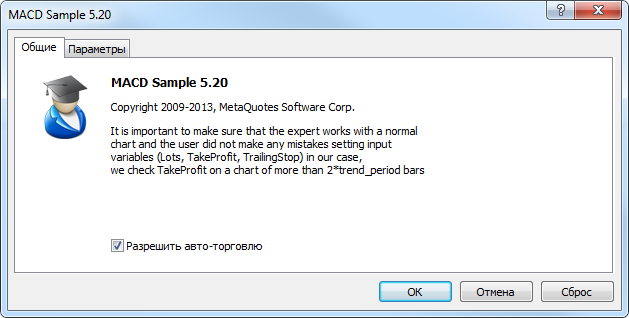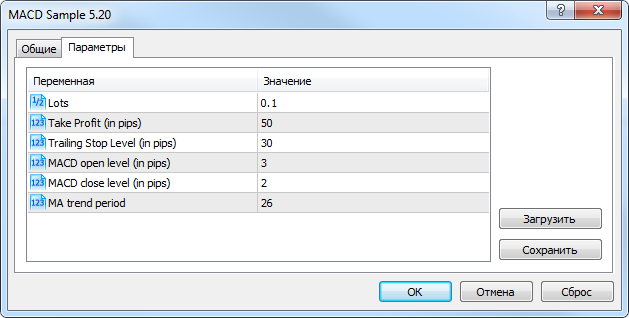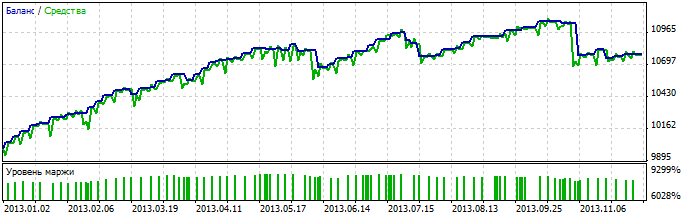# MACD 样本 - MetaTrader 5EA

4048

2014.06.13 13:57

2016.11.22 07:33

1 EA 属性

1.1. EA 属性

```//+------------------------------------------------------------------+
//|                                                  MACD Sample.mq5 |
//|                   Copyright 2009-2013, MetaQuotes Software Corp. |
//|                                              https://www.mql5.com |
//+------------------------------------------------------------------+
#property version     "5.20"
#property description "重要的是确认 EA 可以工作在正常的"
#property description "图表中，并且用户没有造成任何输入"
#property description "变量 (手数, 止盈, 尾随止损) 的错误,"
#property description "我们检查图表上的止盈大于 2*trend_period 柱线"```1.2. 包括文件

• SymbolInfo.mqh (CSymbolInfo - 交易工具属性的工具类);
• PositionInfo.mqh (CPositionInfo - 仓位属性的工具类);
• AccountInfo.mqh (CAccountInfo - 帐户属性的工具类)。
```//--- 包括文件

1.3. 输入

```//--- EA 输入参数
input double InpLots          =0.1; // 手数
input int    InpTakeProfit    =50;  // 止盈 (标准点)
input int    InpTrailingStop  =30;  // 尾随止损级别 (标准点)
input int    InpMACDOpenLevel =3;   // MACD 开仓级别 (标准点)
input int    InpMACDCloseLevel=2;   // MACD 平仓级别 (标准点)
input int    InpMATrendPeriod =26;  // 均线趋势周期```

InpLots - 交易量, InpTakeProfit 和 InpTrailingStop 确定止盈和尾随止损级别。1.4. 全局变量

```int ExtTimeOut=10; // 时间 (单位秒) 交易操作时间间隔
```

```//--- ExtExpert 全局变量
CSampleExpert ExtExpert;```

2. 时间处理函数

2.1. OnInit() 初始化函数

OnInit() 函数在 EA 首次开始运行时被调用一次。通常在 OnInit() 事件处理器中 EA 进行操作准备: 输入参数检查, 指标和参数初始化等。在出现严重错误情况下，当进一步的工作没有了意义，函数退出，返回代码 INIT_FAILED。

```//+------------------------------------------------------------------+
//| EA 函数初始化                                                    |
//+------------------------------------------------------------------+
int OnInit(void)
{
//--- 初始化并创建所需的对象
if(!ExtExpert.Init())
return(INIT_FAILED);
//--- 初始化成功
return(INIT_SUCCEEDED);
}
```

2.2. OnTick() 函数

```//+------------------------------------------------------------------+
//| EA 的新即时价格处理函数                                          |
//+------------------------------------------------------------------+
void OnTick(void)
{
static datetime limit_time=0; // 保存最后调用时间 + 过期
//--- 在请求时间未耗尽之前不要操作
if(TimeCurrent()>=limit_time)
{
//--- 检查数据
if(Bars(Symbol(),Period())>2*InpMATrendPeriod)
{
//--- 在调用 Processing() 方法之后 依据 ExtTimeOut 增加 limit_time 的数值
if(ExtExpert.Processing())
limit_time=TimeCurrent()+ExtTimeOut;
}
}
}```

2.3. OnDeInit() 卸载函数

OnDeInit() 当 EA 从图表中删除时会被调用。如果一个程序在操作期间放置了图形对象, 它们可以从图表中删除。

3. CSampleExpert 类

3.1. CSampleExpert 类

```//+------------------------------------------------------------------+
//|  MACD 样本 EA 类例子                                             |
//+------------------------------------------------------------------+
class CSampleExpert
{
protected:
//--- 保护变量 - 类成员再类方法内部可见
CSymbolInfo       m_symbol;                     // CSymbolInfo 类实例
CPositionInfo     m_position;                   // CPositionInfo 类实例
CAccountInfo      m_account;                    // CAccountInfo 类实例
//--- 指标句柄
int               m_handle_macd;                // 这是 MACD 指标句柄
int               m_handle_ema;                 // 这是移动均线句柄
//--- 指标缓存区
double            m_buff_MACD_main[];           // MACD 指标的主线缓存区
double            m_buff_MACD_signal[];         // MACD 指标的信号线缓存区
double            m_buff_EMA[];                 // EMA 指标缓存区
//--- 当前指标数值
double            m_macd_current;
double            m_macd_previous;
double            m_signal_current;
double            m_signal_previous;
double            m_ema_current;
double            m_ema_previous;
//--- 级别 (单位为标准点)
double            m_macd_open_level;
double            m_macd_close_level;
double            m_traling_stop;
double            m_take_profit;

public:
//--- 构造器
CSampleExpert(void);
//--- 析构器
~CSampleExpert(void);
//--- 公有方法可以从类外部调用
//--- 初始化方法
bool              Init(void);
//--- 卸载方法
void              Deinit(void);
//--- 处理方法
bool              Processing(void);
protected:
//--- 保护方法可以在类方法内部存取
bool              InitIndicators(void);
bool              LongClosed(void);
bool              ShortClosed(void);
bool              LongModified(void);
bool              ShortModified(void);
bool              LongOpened(void);
bool              ShortOpened(void);
};```

1. CSampleExpert(void) - 构造器  (当创建类实例时自动调用);
2. ~CSampleExpert(void) - 析构器 (当类实例被删除时自动调用);
3. bool Init(void) - 初始化方法, 准备操作所需的所有数据;
4. void Deinit(void) - 卸载方法;
5. bool Processing(void) - 处理方法。

CSampleExpert 类成员变量声明带有 protected 存取修饰符，仅在 CSampleExpert 类的方法内部可见 (以及子类)。

1. double           m_adjusted_point - 乘数变量，用于校正 3/5-位报价;
3. CSymbolInfo  m_symbol - CSymbolInfo 类实例;
4. CPositionInfo  m_position - СPositionInfo 类实例;
5. CAccountInfo  m_account - CAccountInfo 类实例;
6. int                 m_handle_macd - 保存 MACD 指标 句柄的变量。
7. int                 m_handle_ema - 保存 EMA 指标 句柄的变量;
8. double           m_buff_MACD_main[] - 动态浮点类型数组, 用于请求 MACD 主线数值;
9. double           m_buff_MACD_signal[] - 动态浮点类型数组, 用于请求 MACD 信号线数值;
10. double           m_buff_EMA[] - 动态浮点类型数组, 用于请求 EMA 指标数值;
11. double           m_macd_current - 用于保存当前 MACD 主线数值;
12. double           m_macd_previous - 用于保存之前 MACD 主线数值;
13. double           m_signal_current - 用于保存当前 MACD 信号线数值;
14. double           m_signal_previous - 用于保存之前 MACD 信号线数值;
15. double           m_ema_current - 用于保存当前 EMA 指标数值;
16. double           m_ema_previous - 用于保存之前 EMA 指标数值
17. double           m_macd_open_level,
18. double           m_macd_close_level,
19. double           m_traling_stop,
20. double           m_take_profit - 用于保存价格级别 (输入参数中设置) 将与账户的 m_adjusted_point 相乘。

CSampleExpert 类方法声明带有 protected 存取修饰符:

1. bool  InitCheckParameters(const int digits_adjust) - 输入参数和 EA 参数初始化的正确性检查;
2. bool  InitIndicators(void) - 初始化 (创建 ) MACD移动均线 指标;
3. bool  LongClosed(void) - 如果多头平仓条件满足，返回 true (并关闭一个已开多头);
4. bool  ShortClosed(void) - 如果空头平仓条件满足，返回 true (并关闭一个已开空头);
5. bool  LongModified(void) - 如果已开多头止损位变更条件满足，返回 true (并修改止损价格);
6. bool  ShortModified(void) - 如果已开空头止损位变更条件满足，返回 true (并修改止损价格);
7. bool  LongOpened(void) - 如果多头开仓条件满足，返回 true (并开多头仓位);
8. bool  ShortOpened(void) - 如果空头开仓条件满足，返回 true (并开空头仓位)。

3.2. CSampleExpert 类构造器

```//+------------------------------------------------------------------+
//| CSampleExpert 类构造器                                           |
//+------------------------------------------------------------------+
m_handle_macd(INVALID_HANDLE),
m_handle_ema(INVALID_HANDLE),
m_macd_current(0),
m_macd_previous(0),
m_signal_current(0),
m_signal_previous(0),
m_ema_current(0),
m_ema_previous(0),
m_macd_open_level(0),
m_macd_close_level(0),
m_traling_stop(0),
m_take_profit(0)
{
ArraySetAsSeries(m_buff_MACD_main,true);
ArraySetAsSeries(m_buff_MACD_signal,true);
ArraySetAsSeries(m_buff_EMA,true);
}```

3.3. CSampleExpert 类析构器

```//+------------------------------------------------------------------+
//| CSampleExpert 类析构器                                           |
//+------------------------------------------------------------------+
CSampleExpert::~CSampleExpert(void)
{
}```

3.4. CSampleExpert 类的 Init 方法

```//+------------------------------------------------------------------+
//| 初始化并验证输入参数                                             |
//+------------------------------------------------------------------+
bool CSampleExpert::Init(void)
{
//--- 设置通用属性
m_symbol.Name(Symbol());              // 品种symbol
//--- 校正账户 3/5-位报价
if(m_symbol.Digits()==3 || m_symbol.Digits()==5)
//--- 设置滑点 3 点
//---
return(false);
if(!InitIndicators())
return(false);
//--- 全部成功
return(true);
}```

3.5. CSampleExpert 类的 InitCheckParameters

```//+------------------------------------------------------------------+
//| 检查输入参数                                                     |
//+------------------------------------------------------------------+
{
//--- 检查止盈级别正确性
{
printf("止盈必须大于 %d",m_symbol.StopsLevel());
return(false);
}
//--- 检查尾随止损级别正确性
{
printf("尾随止损必须大于 %d",m_symbol.StopsLevel());
return(false);
}
//--- 检查交易量正确性
if(InpLots<m_symbol.LotsMin() || InpLots>m_symbol.LotsMax())
{
printf("手数范围必须从 %f 到 %f",m_symbol.LotsMin(),m_symbol.LotsMax());
return(false);
}
if(MathAbs(InpLots/m_symbol.LotsStep()-MathRound(InpLots/m_symbol.LotsStep()))>1.0E-10)
{
printf("手数不符合递增值 %f",m_symbol.LotsStep());
return(false);
}
//--- 如果 Take Profit<=Trailing Stop，显示警告
if(InpTakeProfit<=InpTrailingStop)
printf("警告: 尾随止损必须小于止盈");
//--- 全部成功
return(true);
}```

3.6. CSampleExpert 类的 InitIndicators() 方法

```//+------------------------------------------------------------------+
//| 指标初始化方法                                                   |
//+------------------------------------------------------------------+
bool CSampleExpert::InitIndicators(void)
{
//--- 创建 MACD 指标
if(m_handle_macd==INVALID_HANDLE)
if((m_handle_macd=iMACD(NULL,0,12,26,9,PRICE_CLOSE))==INVALID_HANDLE)
{
printf("创建 MACD 指标错误");
return(false);
}
//--- 创建 EMA 指标
if(m_handle_ema==INVALID_HANDLE)
if((m_handle_ema=iMA(NULL,0,InpMATrendPeriod,0,MODE_EMA,PRICE_CLOSE))==INVALID_HANDLE)
{
printf("创建 EMA 指标错误");
return(false);
}
//--- 全部成功
return(true);
}```

3.7. CSampleExpert 类的 LongClosed() 方法

```//+------------------------------------------------------------------+
//| 检查多头的平仓条件                                               |
//+------------------------------------------------------------------+
bool CSampleExpert::LongClosed(void)
{
bool res=false;
//--- 持仓将被平仓?
if(m_macd_current>0)
if(m_macd_current<m_signal_current && m_macd_previous>m_signal_previous)
if(m_macd_current>m_macd_close_level)
{
//--- 平仓
printf("多头仓位 %s 将被平仓",Symbol());
else
res=true;
}
//--- 返回结果
return(res);
}```

1. m_macd_current>0 - MACD 指标主线的当前数值为正 (MACD 直方图高于零轴);
2. m_macd_current<m_signal_current && m_macd_previous>m_signal_previous - MACD 指标主线下穿信号线。
3. m_macd_current>m_macd_close_level - MACD 指标主线的当前数值大于 m_macd_close_level。

3.8. CSampleExpert 类 ShortClosed() 方法

```//+------------------------------------------------------------------+
//| 检查空头平仓条件                                                 |
//+------------------------------------------------------------------+
bool CSampleExpert::ShortClosed(void)
{
bool res=false;
//--- 持仓将被平仓?
if(m_macd_current<0)
if(m_macd_current>m_signal_current && m_macd_previous<m_signal_previous)
if(MathAbs(m_macd_current)>m_macd_close_level)
{
//--- 平仓
printf("空头持仓 %s 将被平仓",Symbol());
else
res=true;
}
//--- 返回结果
return(res);
}```

1. m_macd_current<0 - MACD 指标主线的当前数值为负 (MACD 直方图低于零轴).
2. m_macd_current>m_signal_current && m_macd_previous<m_signal_previous - MACD 指标主线上穿信号线。
3. MathAbs(m_macd_current)>m_macd_close_level - MACD 指标主线的当前数值大于 m_macd_close_level。

3.9. CSampleExpert 类 LongModified() 方法

```//+------------------------------------------------------------------+
//| 检查多头修改条件                                                 |
//+------------------------------------------------------------------+
bool CSampleExpert::LongModified(void)
{
bool res=false;
//--- 检查是否需要尾随止损
if(InpTrailingStop>0)
{
{
double sl=NormalizeDouble(m_symbol.Bid()-m_traling_stop,m_symbol.Digits());
double tp=m_position.TakeProfit();
if(m_position.StopLoss()<sl || m_position.StopLoss()==0.0)
{
//--- 修改持仓的止损和止盈
printf("多头持仓 %s 将被修改",Symbol());
else
{
printf("修改参数 : SL=%f,TP=%f",sl,tp);
}
res=true;
}
}
}
//--- 返回结果
return(res);
}```

3.10. CSampleExpert 类 ShortModified 方法

```//+------------------------------------------------------------------+
//| 检查空头持仓修改条件                                             |
//+------------------------------------------------------------------+
bool CSampleExpert::ShortModified(void)
{
bool   res=false;
//--- 检查是否需要尾随止损
if(InpTrailingStop>0)
{
{
double tp=m_position.TakeProfit();
if(m_position.StopLoss()>sl || m_position.StopLoss()==0.0)
{
//--- 修改持仓的止损和止盈
printf("空头持仓 %s 将被修改",Symbol());
else
{
printf("修改参数 : SL=%f,TP=%f",sl,tp);
}
res=true;
}
}
}
//--- 返回结果
return(res);
}```

3.11. CSampleExpert 类 LongOpened() 方法

```//+------------------------------------------------------------------+
//| 检查多头开仓条件                                                 |
//+------------------------------------------------------------------+
bool CSampleExpert::LongOpened(void)
{
bool res=false;
//--- 检查多头开仓条件
if(m_macd_current<0)
if(m_macd_current>m_signal_current && m_macd_previous<m_signal_previous)
if(MathAbs(m_macd_current)>(m_macd_open_level) && m_ema_current>m_ema_previous)
{
double tp   =m_symbol.Bid()+m_take_profit;
//--- 检查剩余保证金
printf("资金不足。剩余保证金 = %f",m_account.FreeMargin());
else
{
//--- 开多头仓位
printf("仓位 %s 将被开仓",Symbol());
else
{
printf("开仓参数 : price=%f,TP=%f",price,tp);
}
}
res=true;
}
//--- 返回结果
return(res);
}```

1. m_macd_current<0 - MACD 指标主线的当前数值为负 (MACD 直方图低于零轴);
2. m_macd_current>m_signal_current && m_macd_previous<m_signal_previous - MACD 指标主线上穿信号线;
3. MathAbs(m_macd_current)>m_macd_open_level - MACD 指标主线的当前数值大于 m_macd_open_level;
4. m_ema_current>m_ema_previous - ema grows.

3.12. CSampleExpert 类 ShortOpened 方法

```//+------------------------------------------------------------------+
//| 检查空头开仓条件                                                 |
//+------------------------------------------------------------------+
bool CSampleExpert::ShortOpened(void)
{
bool res=false;
//--- 检查空头开仓条件
if(m_macd_current>0)
if(m_macd_current<m_signal_current && m_macd_previous>m_signal_previous)
if(m_macd_current>(m_macd_open_level) && m_ema_current<m_ema_previous)
{
double price=m_symbol.Bid();
//--- 检查剩余保证金
if(m_account.FreeMarginCheck(Symbol(),ORDER_TYPE_SELL,InpLots,price)<0.0)
printf("资金不足。剩余保证金 = %f",m_account.FreeMargin());
else
{
//--- 开空头仓位
printf("仓位 %s 将被开仓",Symbol());
else
{
printf("开仓参数 : price=%f,TP=%f",price,tp);
}
}
res=true;
}
//--- 返回结果
return(res);
}```

1. m_macd_current>0 - MACD 指标主线的当前数值为正 (MACD 直方图高于零轴);
2. m_macd_current<m_signal_current && m_macd_previous>m_signal_previous - MACD 指标主线下穿信号线。
3. m_macd_current>m_macd_open_level - MACD 指标主线的当前数值大于 m_macd_open_level;
4. m_ema_current<m_ema_previous - ema falls.

3.13CSampleExpert 类 Processing() 方法

```//+------------------------------------------------------------------+
//| 如果处理过任何持仓，主函数返回 true                              |
//+------------------------------------------------------------------+
bool CSampleExpert::Processing(void)
{
//--- 更新报价
if(!m_symbol.RefreshRates())
return(false);
//--- 更新指标数值
if(BarsCalculated(m_handle_macd)<2 || BarsCalculated(m_handle_ema)<2)
return(false);
if(CopyBuffer(m_handle_macd,0,0,2,m_buff_MACD_main)  !=2 ||
CopyBuffer(m_handle_macd,1,0,2,m_buff_MACD_signal)!=2 ||
CopyBuffer(m_handle_ema,0,0,2,m_buff_EMA)         !=2)
return(false);
//--- 简化指标工作并加快存取
//--- 当前指标数值保存于内部变量 (类成员)
m_macd_current   =m_buff_MACD_main;
m_macd_previous  =m_buff_MACD_main;
m_signal_current =m_buff_MACD_signal;
m_signal_previous=m_buff_MACD_signal;
m_ema_current    =m_buff_EMA;
m_ema_previous   =m_buff_EMA;
//--- 正确入场是重要的, 但正确退出更重要
//--- 首先检查是否有已开持仓
if(m_position.Select(Symbol()))
{
{
//--- 如果必要, 我们尝试平掉或修改多头仓
if(LongClosed())
return(true);
if(LongModified())
return(true);
}
else
{
//--- 如果必要, 我们尝试平掉或修改空头仓
if(ShortClosed())
return(true);
if(ShortModified())
return(true);
}
}
//--- 无开仓
else
{
//--- 若必要，检查开多头仓位条件并开仓
if(LongOpened())
return(true);
//--- 若必要，检查开空头仓位条件并开仓
if(ShortOpened())
return(true);
}
//--- 不处理仓位退出
return(false);
}```

CSampleExpert 类 Processing() 方法是 EA 的方法。该 Processing() 方法在 OnTick() 时间处理器中调用, 并且监控方法调用的时间间隔 (不小于 ExtTimeOut 秒) (第 2.2 部分)。

4. 回测MultiT3_TRIXx7SignalBackgroundCandle_T3_TRIX_HTFExp_T3_TRIXExp_RSIOMA_V2